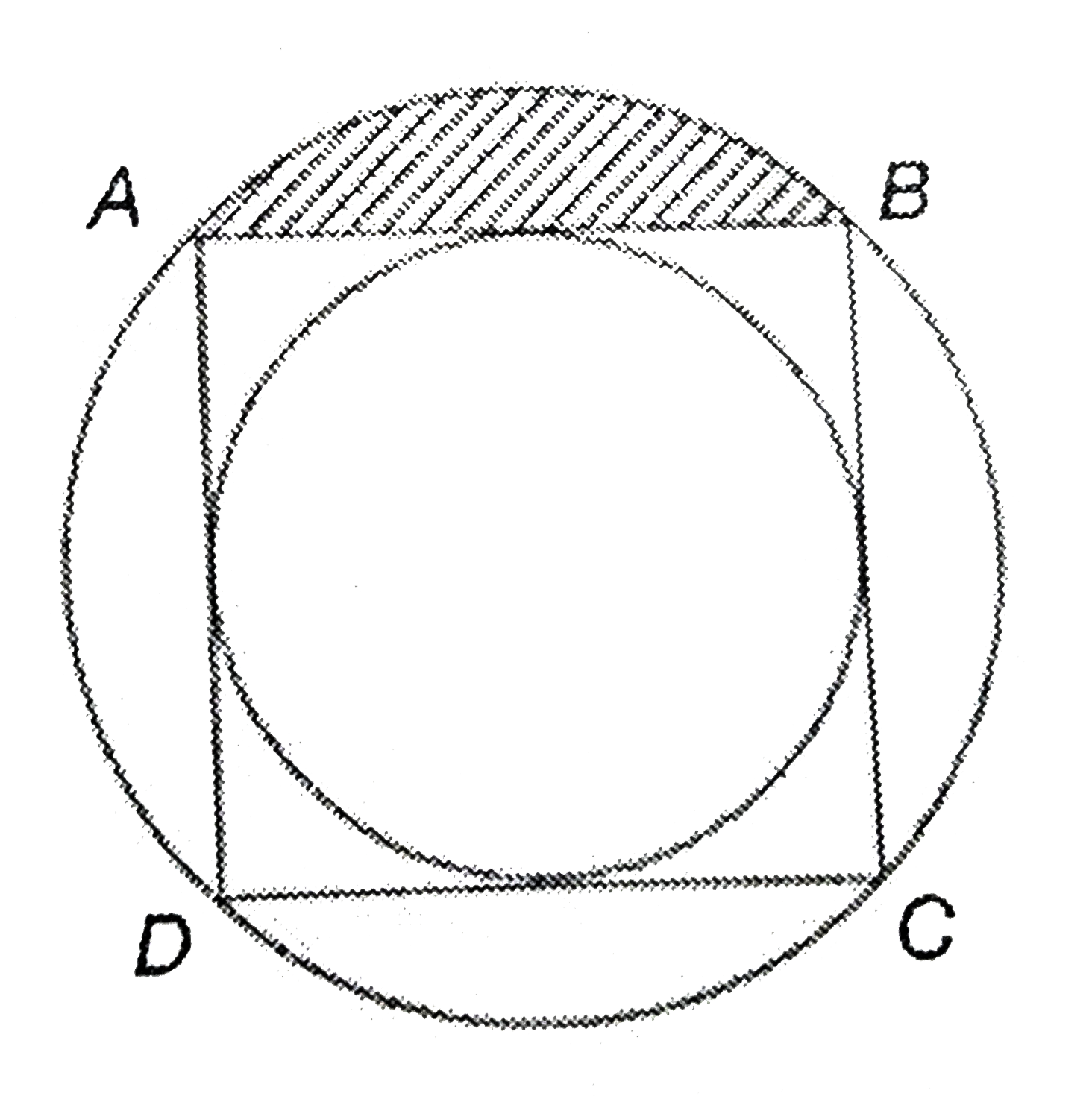# Pls explain to me in detail.

+1 vote
77 views

closed

In the figure, a circle is inscribed in a square ABCD and a square is inscribed by a circle. If the radius of the smaller circle is r cm, then the area of the shaded region(in cm2) is:-(a) {(π - 2)/4}r2

(b) {(3π - 4)/2}r2

(c)  {(π + 2)/4}r2

(d) {(π - 2)/2}r2

by (415 points)
edited byLet O be the centre of both the concentric circles. Now, given that radius of smaller circles is r. Then radius of bigger circle will be OA = √2r (By pythgoras theorem).

Area of sector AOB - Area of triangle AOB

90º/360°×π×(Radius of larger circle)² - 1/2×base×height (Notice that the angle being subtended at centre is 90° because we know that diagonals of a square bisect each other at 90°)

1/4π(√2r)²-1/2√2r√2r

(π2r²/4)-r²

(π2r²-4r²)/4

2r²{(π-2)/4}

{(π-2)/2}r²

Therefore, option (D) is correct.

+1 vote